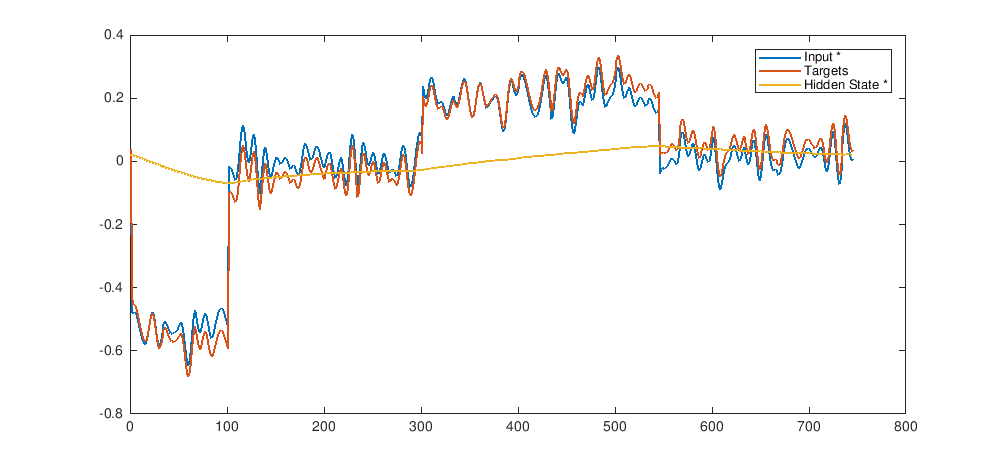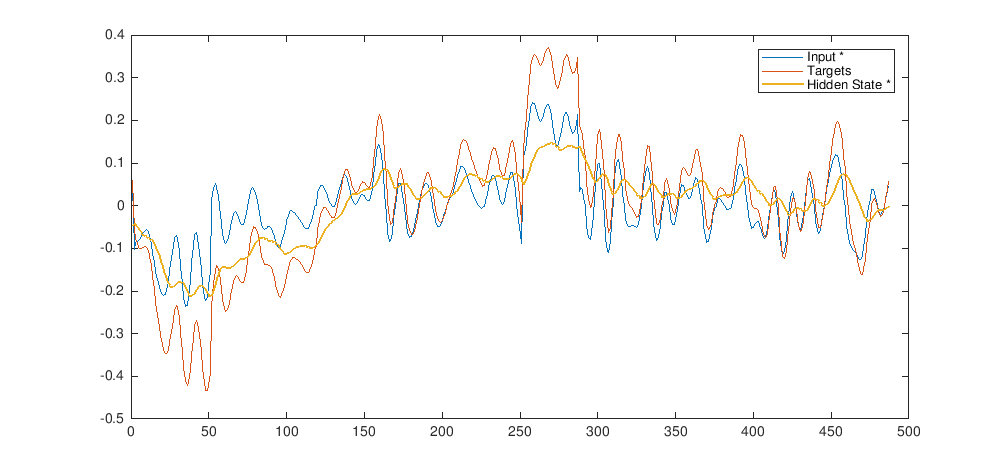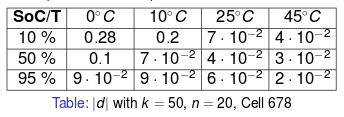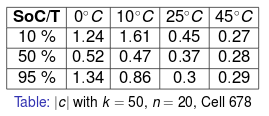These considerations suggest a connection between the hidden variable and the over-potential. Indeed, we can see for high temperatures and SoCs that the hidden variable is low and the non-linearity redundant, leading to the unnecessary cost of a non-linear optimisation. These considerations are consistent with the Butler-Volmer equation: in the limiting case of low over-potential, the over-potential is linearly proportional to the current. On the other hand, for low SoCs and temperatures the hidden variable is better approximated by non-linear functions, in accordance with the Tafel relation. Subspace methods and neural networks also show an inverse proportionality between temperature and the multiplicative parameters$d$ and$c$, which appear in both the methods (for non-linear neural networks it corresponds to$c_1$ and$c_2$).